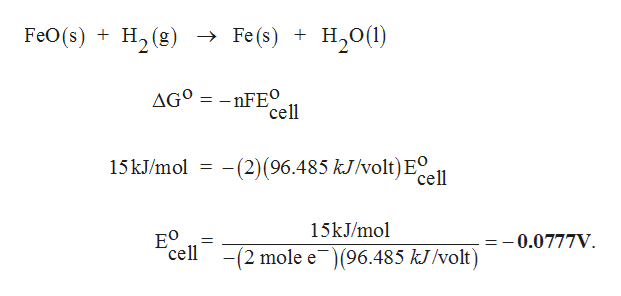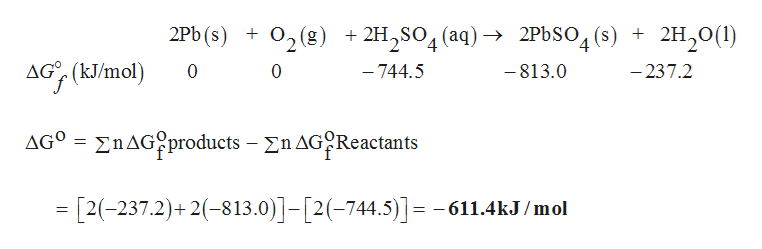# Starting with the standard free energies of formation from the following table, calculate the values of ΔG° and E°cell of the following reactions. SubstanceΔΔG°f (kJ/mol)FeO(s)-255.2H2(g)0Fe(s)0H2O(l)-237.2Pb(s)0O2(g)0H2SO4(aq)-744.5PbSO4(s)-813.0 FeO(s)+H2​(g)Fe(s)+H2​O(l) ΔΔG° =     kJ     FeO(s)+H2​(g)Fe(s)+H2​O(l) E°cell =     V     2Pb(s)+O2​(g)+2H2​SO4​(aq)2PbSO4​(s)+2H2​O(l)  ΔΔG° =     kJ      Pb(s)+O2​(g)+2H2​SO4​(aq)2PbSO4​(s)+2H2​O(l)  E°cell =     V

Question
53 views
Starting with the standard free energies of formation from the following table, calculate the values of ΔG° and cell of the following reactions.

 Substance ΔΔG°f (kJ/mol) FeO(s) -255.2 H2(g) 0 Fe(s) 0 H2O(l) -237.2 Pb(s) 0 O2(g) 0 H2SO4(aq) -744.5 PbSO4(s) -813.0

FeO(s)+H2​(g)Fe(s)+H2​O(l)
ΔΔG° =     kJ

FeO(s)+H2​(g)Fe(s)+H2​O(l)
E°cell =     V

2Pb(s)+O2​(g)+2H2​SO4​(aq)2PbSO4​(s)+2H2​O(l)
ΔΔG° =     kJ

Pb(s)+O2​(g)+2H2​SO4​(aq)2PbSO4​(s)+2H2​O(l)
cell =     V

check_circle

Step 1

For reaction (1),

The enthalpy change is calculated for the given reaction as follows,

Step 2

The standard cell potential is calculated using,help_outlineImage TranscriptioncloseFeO(s) H (g) » Fe(s) + H,0(1) Н. AGO nFEO cell -(2) (96.485 kJ/volt) EQ 15 kJ/mol cell 15kJ/mol ЕO cell =-0.0777V = -(2 mole e(96.485 kJ/volt) fullscreen
Step 3

For reaction (2),

The enthalpy change is calculat...help_outlineImage Transcriptionclose2Pb (s) O2(g) + 2H2SO4(aq)- 2P6SO4(s) 2H20(1) AG (kJ/mol 0 0 -744.5 -813.0 -237.2 nAGproducts - n AGReactants AGo =[2(-237.2)+2813.0)]-[2(-744.5)] = -611.4kJ /mol fullscreen

### Want to see the full answer?

See Solution

#### Want to see this answer and more?

Solutions are written by subject experts who are available 24/7. Questions are typically answered within 1 hour.*

See Solution
*Response times may vary by subject and question.
Tagged in

### Chemistry